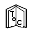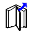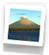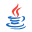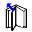## X3D Example Archives: X3D4AM, X3D for Advanced Modeling, Geometric ShapesThis chapter illustrates design patterns and best practices for authoring different geometries through a collection of interesting 3D shapes. The open-source Meshlab and X3D-Edit authoring tools helped create many of these examples. Tools of interest for generating mathematical shapes include:
 Wikipedia: Platonic solid and Geodesic grid Wolfram Mathworld: Geometry X3D-Edit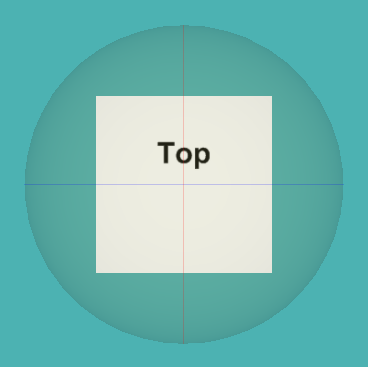32 X3D Models       X3D Model Descriptions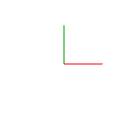Axis Lines RGB AxisLinesRGB shows 3 red-green-blue (RBB) perpendicular lines suitable as X-Y-Z axes.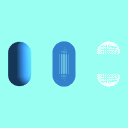Capsule Comparison CapsuleComparison shows three capsules, using polygons/lines/points rendering, each a hollow cylinder with hemispherical end caps. These models were created by CapsuleGenerator.x3d (producing X3D source on the console) with 36 numberOfPoints around horizontal perimeter, 10 vertical levels, radius 0.5 and total height of 2.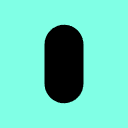Capsule Generator Capsule has top and bottom hemispheres with connected sides, with adjustable parameters in the contained Script fields. A Script node computes all coordIndex and Coordinate point values. X3D source for the produced capsule also appears on the player console.Catenary A catenary is the curve that an idealized hanging chain or cable assumes under its own weight when supported only at its ends. The curve has a U-like shape, superficially similar in appearance to a parabola, but it is not a parabola: it is a (scaled, rotated) graph of the hyperbolic cosine.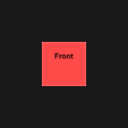Cube With Labeled Sides Cube-shaped test model with faces on each side individually labeled.Cylinder Indexed Face Set Construct a Cylinder using IndexedFaceSet. Top and bottom 24-point polygon (circle) created by X3D-Edit, sides computed manually.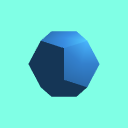Dodecahedron Dodecahedron is a 12-sided polyhedron with 30 edges, 20 vertices and 12 pentagonal faces. A regular dodecahedron is a Platonic solid.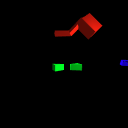Extrusion Edge Cases Scene incorporating extrusion edge casesExtrusion Examples Test Extrusion examples for testing and debugging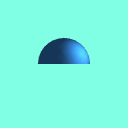Hemisphere Hemisphere is a half sphere. Sphere tessellation algorithms might be insufficient since equatorial triangles may not align with the plane. The original tool or algorithm producing this mesh is not known, unfortunately.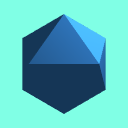Icosahedron Icosahedron is a polyhedron with 12 vertices and, 20 faces, where a regular icosahedron is a Platonic solid. All faces are regular (equilateral) triangles. In comparison to the other icosahedra examples, this this default Icosahedron can be considered at level 0, meaning that no further subdivision has occurred from the platonic solid.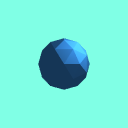Icosahedron Subdivision Level 1 Icosahedron is a polyhedron with twenty faces, subdivided to level 1, where all 42 vertices and 80 faces produce regular (equilateral) triangles.Icosahedron Subdivision Level 2 Icosahedron is a polyhedron with twenty faces, subdivided to level 2, where all 162 vertices and 320 faces produce regular (equilateral) triangles.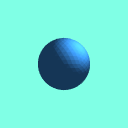Icosahedron Subdivision Level 3 Icosahedron is a polyhedron with twenty faces, subdivided to level 3, where all 642 vertices and 1280 faces produce regular (equilateral) triangles.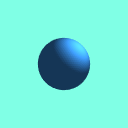Icosahedron Subdivision Level 4 Icosahedron is a polyhedron with twenty faces, subdivided to level 4, where all 2562 vertices and 3366 faces produce regular (equilateral) triangles.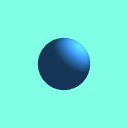Icosahedron Subdivision Level 5 Icosahedron is a polyhedron with twenty faces, subdivided to level 5, where all 10242 vertices and 20480 faces produce regular (equilateral) triangles.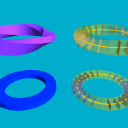Mobius Extrusion Mobius (or Mobius) is a surface with only one side and only one boundary component.Octahedron Octahedron is an 8-sided polyhedron with 6 vertices, 8 triangular faces and 12 edges. A regular octahedron contains equilateral triangles and is a Platonic solid. An Octahedron is equivalent to two contiguous Pyramids. The dual of the Octahedron is the cube.Olympic Rings Olympic Rings built for authoring course MV3204 using regulation colors, each approximately 5m radius. Debugging efforts showed difficulties with concave crossSection, important to set convex=false for proper rendering.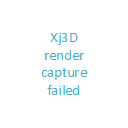Olympic Rings Blender Olympic Rings built using Blender for comparison.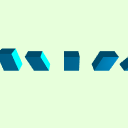Parallelepiped Examples Example use of external prototype declaration for Parallelepiped, which is a 3D figure formed by six parallelograms.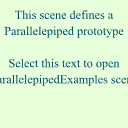Parallelepiped Prototype Reusable prototype for creating a Parallelepiped, which is a 3D figure formed by six parallelograms; also sometimes referred to as a rhomboid.Rounded Rectangle 2D RoundedRectangle2D shows and IndexedFaceSet shape for a flat RoundedRectangle.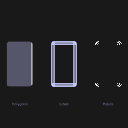Rounded Rectangle Comparison RoundedRectangleComparison shows three RoundedRectangle shapes using polygons/lines/points rendering. Note that reuse of RoundedRectangle geometry requires only Interchange profile, but Text requires Immersive profile.Rounded Rectangle Primitives Illustrates how to build rounded rectangle using only primitive nodes.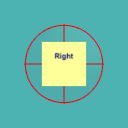Sphere With Three Circumference Rings Semitransparent Sphere with circumference circles on x-y-z planes to facilitate user rotation of an object, suitable for use as rotation widget. Labeled box also inserted as Inline.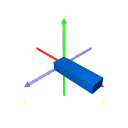Stair Step Prototype Build one or more stair steps, in a straight lineTeapot A watertight (solid) Utah Teapot Model converted from obj format.Teapots Four watertight (solid) Utah Teapot Models converted from obj format.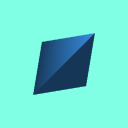Tetrahedron Tetrahedron is an 8-sided pyramidal polyhedron with 4 vertices, 4 triangular faces and 6 edges. A regular tetrahedron contains equilateral triangles and is a Platonic solid. Tetrahedron is the simplest of all the ordinary convex polyhedra and the only one that has fewer than 5 faces. Tetrahedron is also known as a triangular pyramid.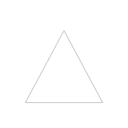Triangle Black Lines Simple Triangle with black lines, illustrating default line rendering.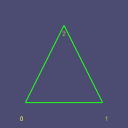Triangle With Numbers Simple Triangle with labeled points, where labels always face the user and stay in place with the triangle.